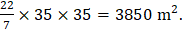# Cost of fencing a circular plot at the rate of Rs 15 per meter is Rs. 3300. What will be cost if flooring the pole at the rate of Rs. 100 per square meter?  (a) Rs. 2,22,000 (b) Rs. 3,50,000 (c) Rs. 3,85,000 (d) Cannot be determined

## Question ID - 50520 :- Cost of fencing a circular plot at the rate of Rs 15 per meter is Rs. 3300. What will be cost if flooring the pole at the rate of Rs. 100 per square meter?  (a) Rs. 2,22,000 (b) Rs. 3,50,000 (c) Rs. 3,85,000 (d) Cannot be determined

3537

Circumference =m = 220 m.m.

Area of plot =Cost of flooring =Rs. 385000.

Next Question :
 If, then a), andare in H.P. b)andare in A.P. c)d)